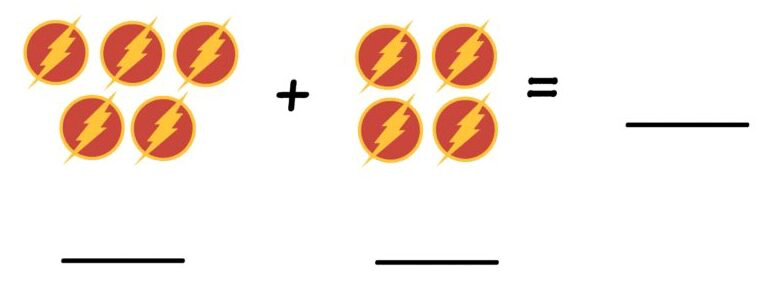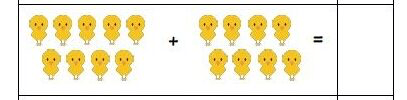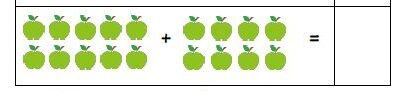Subtraction [-]
Money
Pictures
Multiplication [x]
Word Problems
What comes next?
100

2 + 2 = ?

4

100

4 - 2 = ?

2

100

What 5 coins add up to a nickel?

5 pennies

(1 + 1 + 1 + 1 + 1= 5)

1005

100

1 x 1 = ?

1

100

We had 8 puppies and gave 7 away. How many are left?

1

8 - 7 = 1

100

What number follows zero?

1 (one)

200

6 + 6 = ?

12

200

8 - 2 = ?

6

200

The popsicle costs 25₵. Can you buy it with 2 dimes and 2 pennies?

No

(Another 3₵ is needed.)

2006

(4 + 2 = 6)

200

2 x 2 = ?

4

200

My shirt had 8 buttons, but 2 fell off. How many buttons are left?

6

(8 - 2 = 6)

200

Counting by twos, what number comes next:

5, 7, 9, .......?

11  (eleven)

300

3 + 7 = ?

10

300

7 - 4 = ?

3

300

Jose had 50₵. He spent 30₵ on candy and 20₵ on gum. How much is left?

0₵ (Nothing)

(50 - 30 = 20

20 - 20 = 0)

3009

(5 +  4 =9)

300

3 x 3 = ?

9

300

If you have 2 sisters and 2 brothers, how many children are in your family?

(2 + 2 + 1 = 5)

(Did you remember to count yourself?)

300

What number comes right after 49?

50 (fifty)

400

2 + 3 + 5 = ?

10

400

12 - 5 = ?

7

400

You have 60₵ and you add 2 dimes. How much do you have now?

80₵

(60 + 10 + 10 = 80)

40017

(9 + 8 = 17)

400

4 x 4 = ?

16

400

John repaired 4 pairs of boots. How many boots did he fix in all?

8

(2 + 2 + 2 + 2 =  8)

400

What number comes right after 89?

90 (ninety)

500

10 + 10 = ?

20

500

20 - 10 = ?

10

500

The stickers cost 40₵. Can you buy them with 1 quarter and 5 pennies?

No

(Another 10₵ is needed.)

50018

(10 + 8 = 18)

500

5 x 5 = ?

25

500

Nathan has 5 inches of String. There are 12 inches in 1 foot.

How many more inches do you need to make 1 foot?

7 inches

12 - 5 = 7

(5 + 7 = 12 inches, or 1 foot)

500

Counting by tens, what number comes next:

10, 20, 30, ......?

40 (forty)

Click to zoom